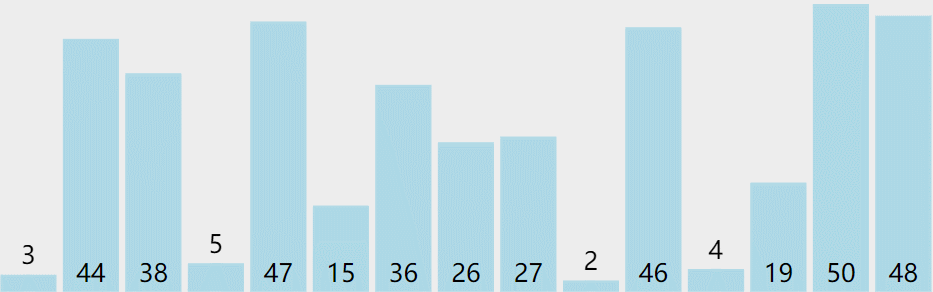﻿ 选择排序 ( 直接选择排序 堆排序 )

### 选择排序 ( 直接选择排序 堆排序 )

2021-11-25 6:39:59
##### 1、直接选择排序

🔑 核心思想 🔑

每一次从待排序的数据元素中选出最小（或最大）的一个元素，存放在序列的起始位置，直到全部待排序的数据元素排完 。

❗ 过程：❕

1️⃣ 在元素集合 array[i] - array[n-1] 中选择关键码最大 (小) 的数据元素

2️⃣ 若它不是这组元素中的最后一个(第一个)元素，则将它与这组元素中的最后一个（第一个）元素交换

3️⃣ 在剩余的 array[i] - array[n-2] (array[i+1]–array[n-1]) 集合中，重复上述步骤，直到集合剩余 1 个元素

❗ 直接选择排序的特性总结：❕

1️⃣ 直接选择排序思考非常好理解，但是效率不是很好。实际中很少使用

2️⃣ 时间复杂度：O(N^2) - 最好 / 最坏

3️⃣ 空间复杂度：O(1)

4️⃣ 稳定性：不稳定

❗ 动图演示：❕🧿 实现代码 ：

``````void Swap(int* px, int* py)
{
int temp = *px;
*px = *py;
*py = temp;
}
void SelectSort(int* a, int n)
{
int i = 0;
int begin = 0;
while (begin < n)
{
int mini = begin;
//选最小
for (i = begin; i < n; i++)
{
if (a[i] < a[mini])
{
mini = i;
}
}
//交换
Swap(&a[begin], &a[mini]);
//迭代
begin++;
}
}
``````

🧿 实现 SelectSort 的优化代码 ：

遍厉一遍选出最小的和最大的，然后把最小的放在左边，最大的放在右边

``````void Swap(int* px, int* py)
{
int temp = *px;
*px = *py;
*py = temp;
}
void SelectSortPro(int* a, int n)
{
int i = 0;
int begin = 0, end = n - 1;
while (begin < end)
{
//选最大和最小
int mini = begin, maxi = begin;
for (i = begin; i <= end; i++)
{
if (a[i] > a[maxi])
{
maxi = i;
}
if (a[i] < a[mini])
{
mini = i;
}
}
//交换
Swap(&a[begin], &a[mini]);
//当a数组里第1个元素是最大值时，此时经过上面的Swap，最大值的位置已经更改了，所以需要修正最大值的位置，让下一个Swap正确交换
if (begin == maxi)
{
maxi = mini;
}
Swap(&a[end], &a[maxi]);
//迭代
++begin;
--end;
}
}
``````
##### 2、堆排序

🔑 核心思想 🔑

堆排序 (Heapsort) 是指利用堆积树 (堆) 这种数据结构所设计的一种排序算法，它是选择排序的一种。它是通过堆来进行选择数据。需要注意的是排升序要建大堆，排降序建小堆。
关于堆排序详解请转到 ➡ 仅不到五万字轻松了解二叉树和堆

❗ 堆排序的特性总结：❕

1️⃣ 堆排序使用堆来选数，效率就高了很多。

2️⃣ 时间复杂度：O(N*logN)

3️⃣ 空间复杂度：O(1)

4️⃣ 稳定性：不稳定

❗ 动图演示：❕🧿 实现代码 ：

``````void Swap(int* px, int* py)
{
int temp = *px;
*px = *py;
*py = temp;
}
void AdjustDown(int* a, int n, int parent)
{
int child = parent * 2 + 1;
while (child < n)
{
if (a[child] < a[child + 1] && child + 1 < n)
{
child++;
}
if (a[child] > a[parent])
{
Swap(&a[child], &a[parent]);
parent = child;
child = parent * 2 + 1;
}
else
{
break;
}
}
}
void HeapSort(int* a, int n)
{
//建大堆
int i = 0;
for (i = (n - 1 - 1) / 2; i >= 0; i--)
{